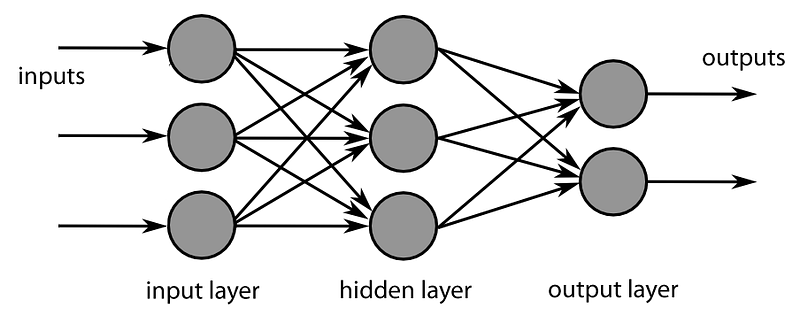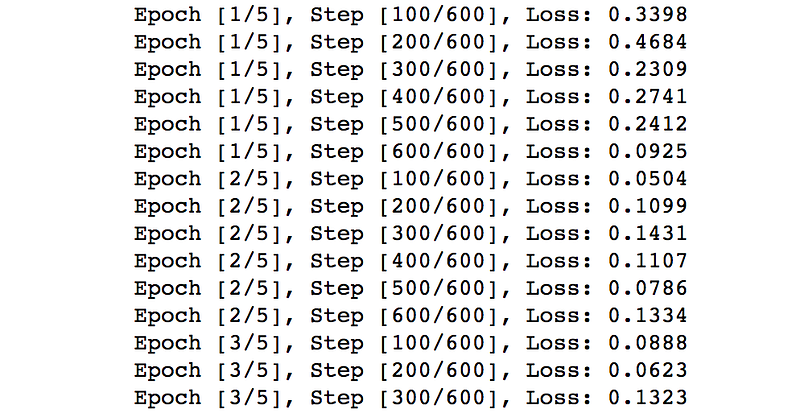#A Simple Starter Guide to Build a Neural Network

This guide serves as a basic hands-on work to lead you through building a neural network from scratch. Most of the mathematical concepts and scientific decisions are left out.comments

By Jeff Hu, Machine Learning EnthusiastYou will be able to program and build a vanilla Feedforward Neural Network (FNN) starting today via PyTorch. Here is the python jupyter codebase for the FNN: https://github.com/yhuag/neural-network-lab

This guide serves as a basic hands-on work to lead you through building a neural network from scratch. Most of the mathematical concepts and scientific decisions are left out. You are free to research more on that part.

### Getting Started

1. Please make sure you have Python and PyTorch installed in your machine:

2. Check the correctness of Python installations by the commands at console:

`python -V`

The output should be Python 3.6.3 or later version

3. Open a repository(folder) and create your first Neural Network file:

```mkdir fnn-tuto
cd fnn-tuto
touch fnn.py```

### Start Writing Codes

All the following codes should be written in the fnn.py file

Import PyTorch

It will load PyTorch into the codes. Great! A well beginning is half done.

Initialize Hyper-parameters

Hyper-parameters are the powerful arguments that are set up upfront and will not be updated along with the training of the neural network.

MNIST is a huge database with tons of handwritten digits (i.e. 0 to 9) aims for the usage of image processing.

Note: We shuffle the loading process of train_dataset to make the learning process independent of data order, but the order of test_loader remains to examine whether we can handle unspecified bias order of inputs.

### Build the Feedforward Neural Network

Now we have our datasets ready. We will start building the neural network. The conceptual illustration can be viewed as below:Feedforward Neural Network Model Structure

The FNN includes two fully-connected layers (i.e. fc1 & fc2) and a non-linear ReLU layer in between. Normally we call this structure 1-hidden layer FNN, without counting the output layer (fc2) in.

By running the forward pass, the input images (x) can go through the neural network and generate a output (out) demonstrating how are the likabilities it belongs to each of the 10 classes. For example, a cat image can have 0.8 likability to a dog class and a 0.3 likability to a airplane class.

Instantiate the FNN

We now create a real FNN based on our structure.

`net = Net(input_size, hidden_size, num_classes)`

Enable GPU

Note: You could enable this line to run the codes on GPU

`# net.cuda()    # You can comment out this line to disable GPU`

Choose the Loss Function and Optimizer

Loss function (criterion) decides how the output can be compared to a class, which determines how good or bad the neural network performs. And the optimizer chooses a way to update the weight in order to converge to find the best weights in this neural network.

```criterion = nn.CrossEntropyLoss()

### Training the FNN Model

This process might takes around 3 to 5 minutes depending on your machine. The detailed explanations are listed as comments (#) in the following codes.

### Testing the FNN Model

Similar to training the neural network, we also need to load batches of test images and collect the outputs. The differences are that:

1. No loss & weights calculation
2. No wights update
3. Has correct prediction calculation

Save the trained FNN Model for future use

We save the trained model as a pickle that can be loaded and used later.

`torch.save(net.state_dict(), ‘fnn_model.pkl’)`

Congrats. You have done building your first Feedforward Neural Network!

### What’s Next

Save and close the file. Start running the file at the console:

`python fnn.py`

You will see the training process going like the following:Thanks for your time and hope you enjoy the tutorial. All the codes can be found here!

Credit: The codes are heavily and thankfully based on yunjey’s great codebase. ❤

Bio: Jeff Hu (Github) is a Taiwanese machine learning enthusiast and blockchain developer. He focuses on Natural Language Processing and Deep Learning at work and is a magician and poet at leisure.

Original. Reposted with permission.

Related: